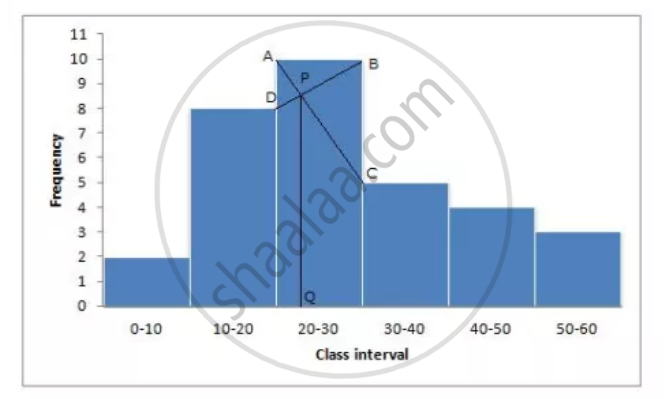Share

# Draw a Histogram and Hence Estimate the Mode for the Following Frequency Distribution: - ICSE Class 10 - Mathematics

ConceptMeasures of Central Tendency - Mean, Median, Mode for Raw and Arrayed Data

#### Question

Draw a histogram and hence estimate the mode for the following frequency distribution:

 Class 0-10 10-20 20-30 30-40 40-50 50-60 Freq 2 8 10 5 4 3

#### Solution

Draw a histogram(1) In the highest rectanglr which represents modal class draw two lines AC aand BD intersecting at P.

(2) From P, draw a perpendicular to x-axis meeting at Q

(3) value of Q is the mode = 23

Is there an error in this question or solution?

#### APPEARS IN

Selina Solution for Selina ICSE Concise Mathematics for Class 10 (2018-2019) (2017 to Current)
Chapter 24: Measure of Central Tendency(Mean, Median, Quartiles and Mode)
24E | Q: 11
Solution Draw a Histogram and Hence Estimate the Mode for the Following Frequency Distribution: Concept: Measures of Central Tendency - Mean, Median, Mode for Raw and Arrayed Data.
S